# Lakhmir Singh Solutions Class 9 Physics Chapter 4 Work and Energy

Work is defined as the force which is required to cause a displacement in the object. It is the product of force acting and the displacement caused in the direction of the force.

 W = F.s

The unit of work is Newton meter of Joule. One joule is defined as the amount of work done by 1N force when 1m displacement is caused. Work done can be either a positive work or a negative work depending on the direction of displacement.

Work done is said to be positive work when both force and displacement are in the same direction. When the force and displacement are in the opposite direction, the work done is said to be negative. But when force and displacement are at an angle of 90 degrees, the work done is said to be zero.

Energy is defined as the ability of an object to do work. Joules is the unit of energy. There are various forms of energy and they are:

Kinetic energy, potential energy, chemical energy, electrical energy, heat energy, and light energy.

Also, according to the law of conservation of energy, energy can neither be created nor be destroyed but can be transformed from one form to another.

## Download PDF Of Lakhmir Singh Physics Class 9 Solutions For Chapter 4 Work and Energy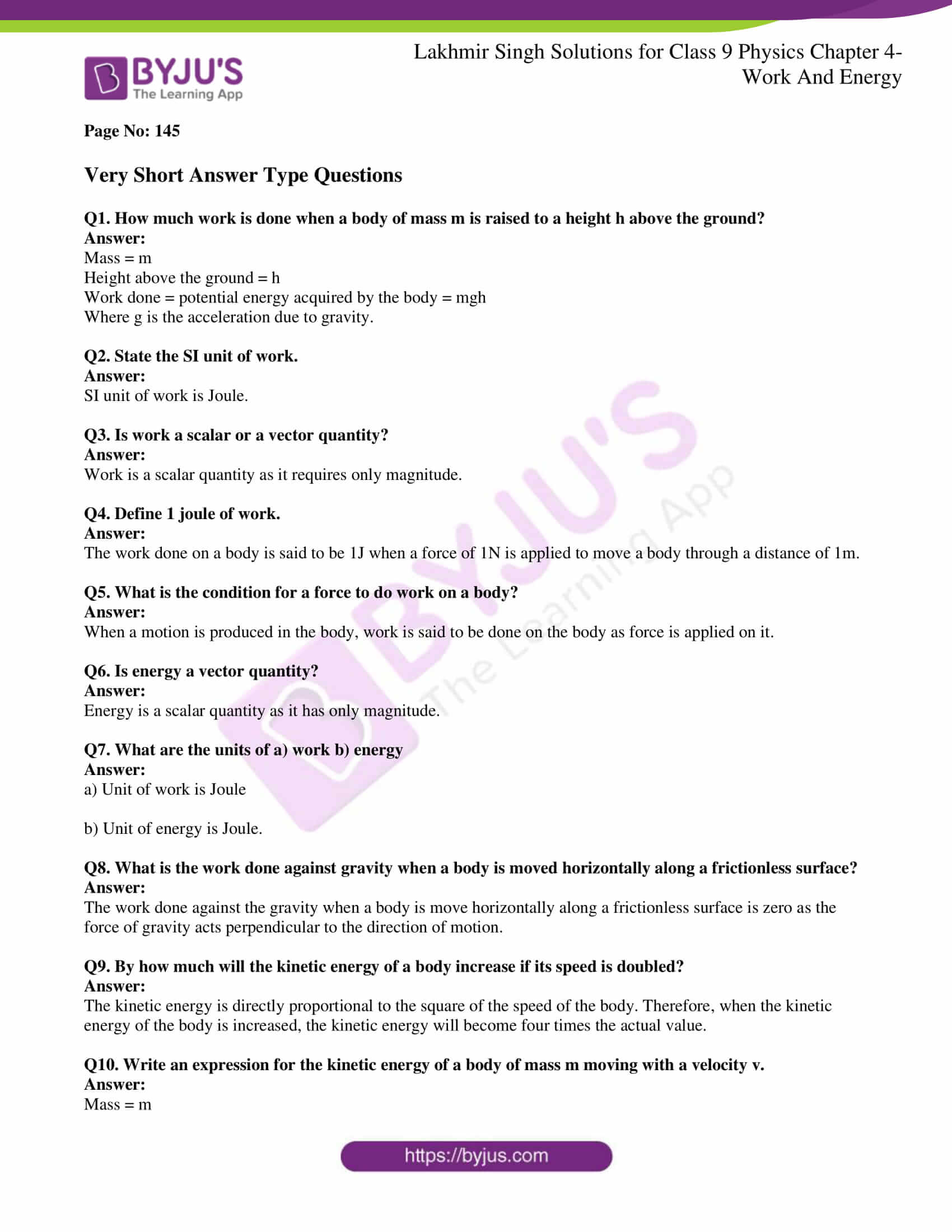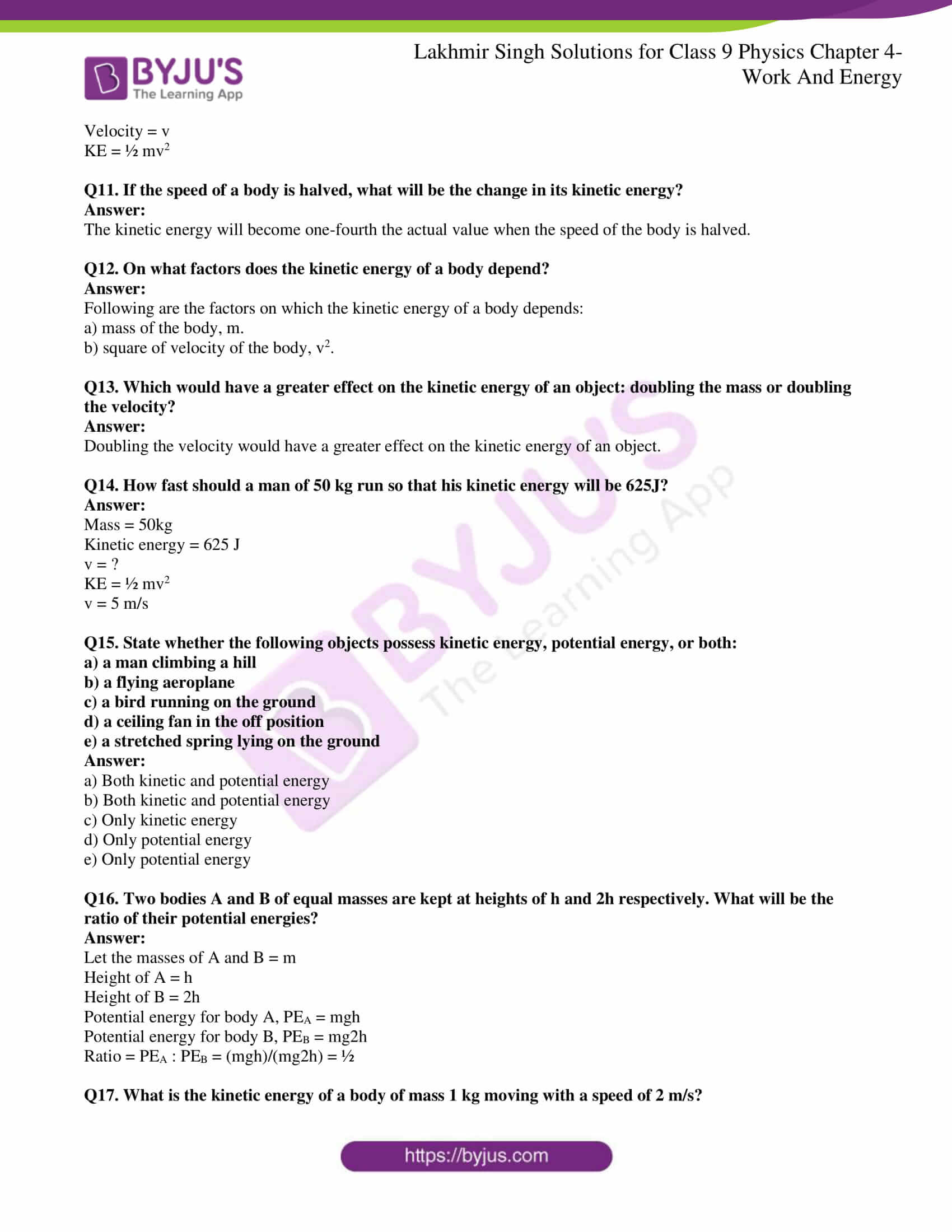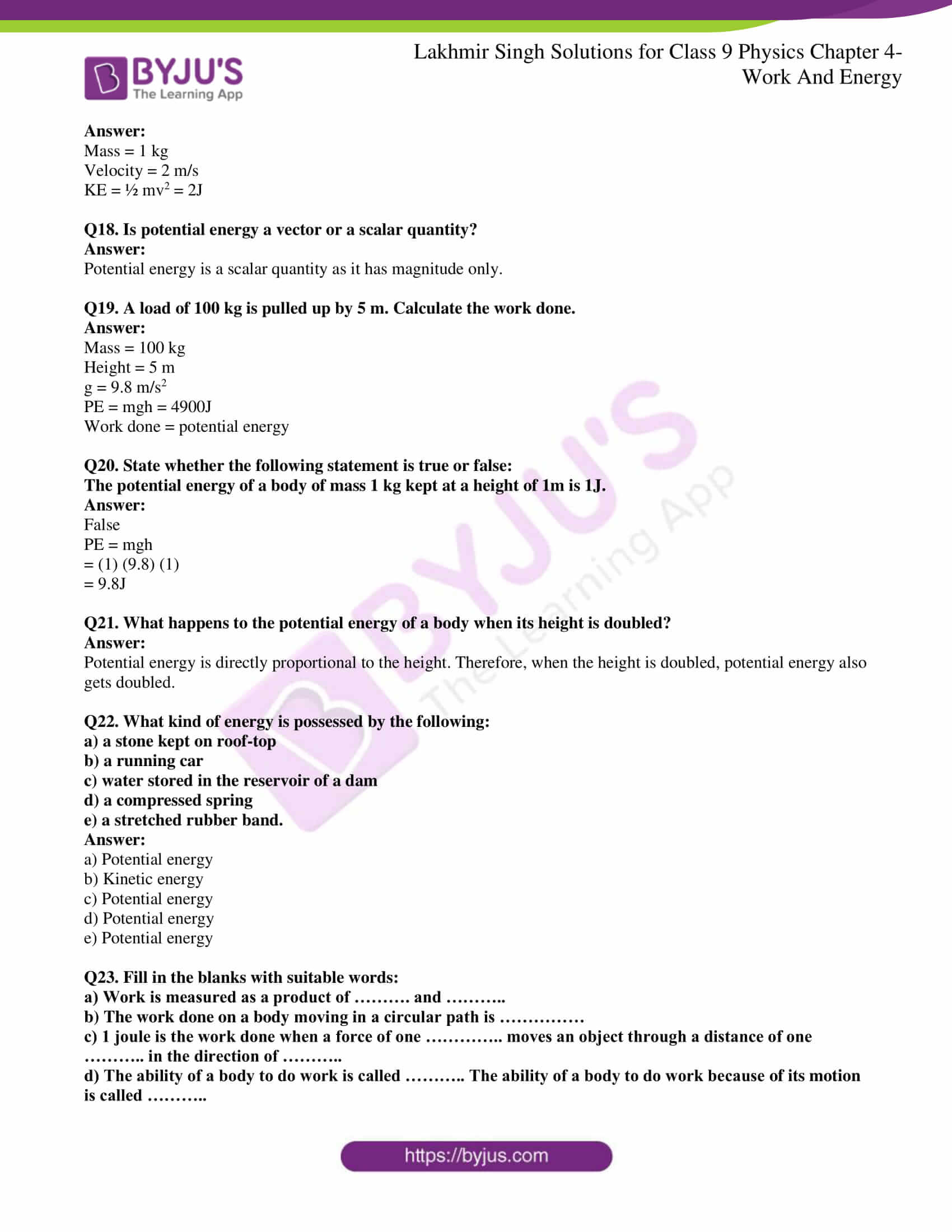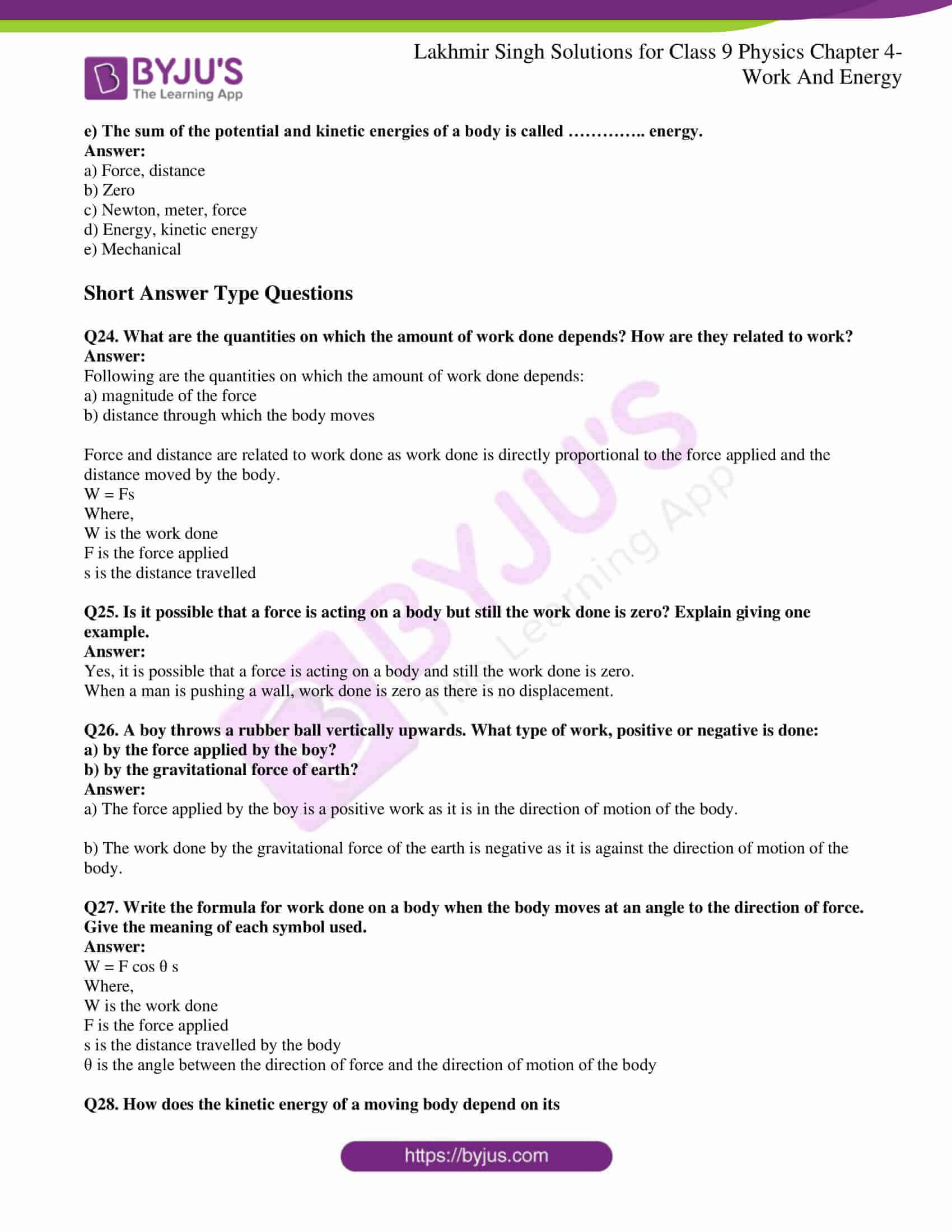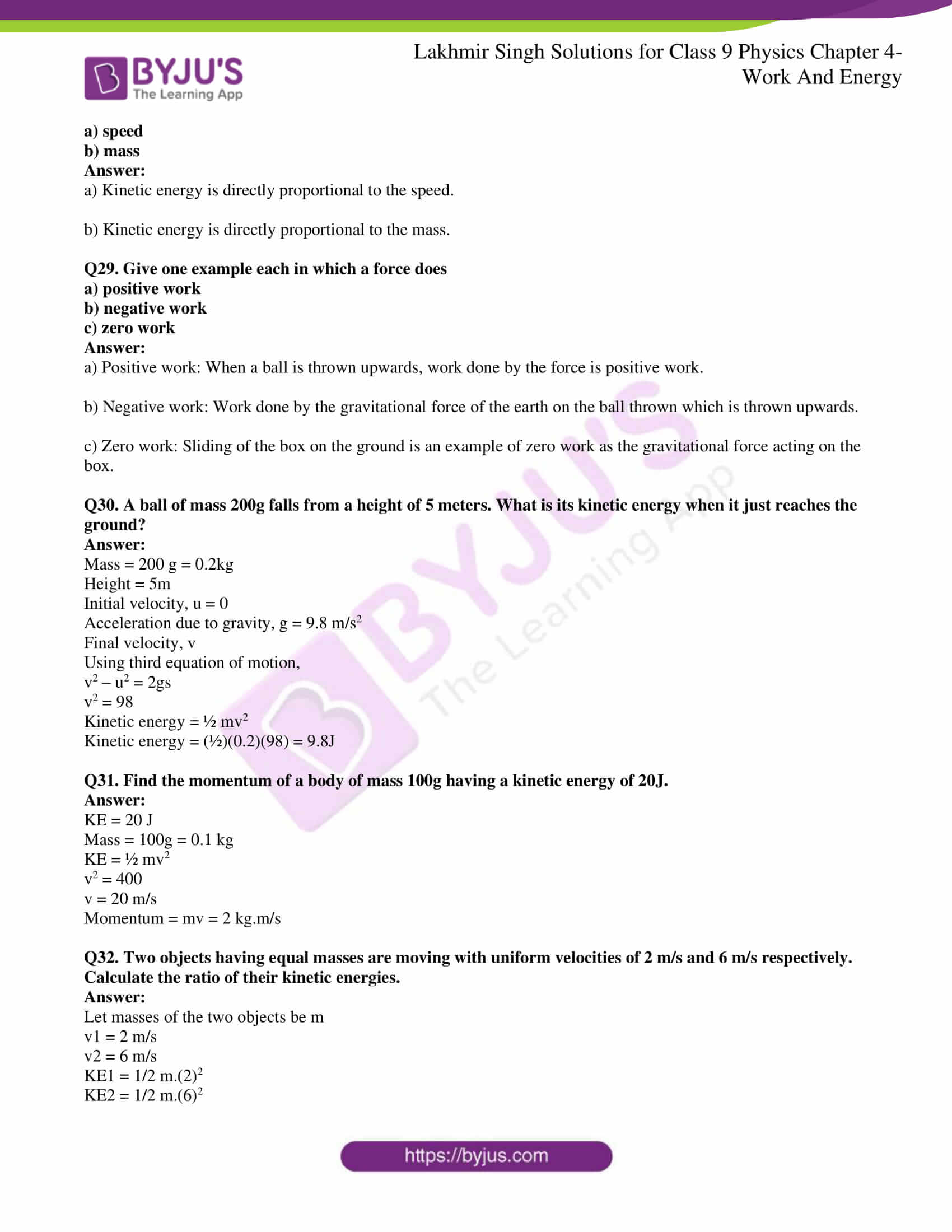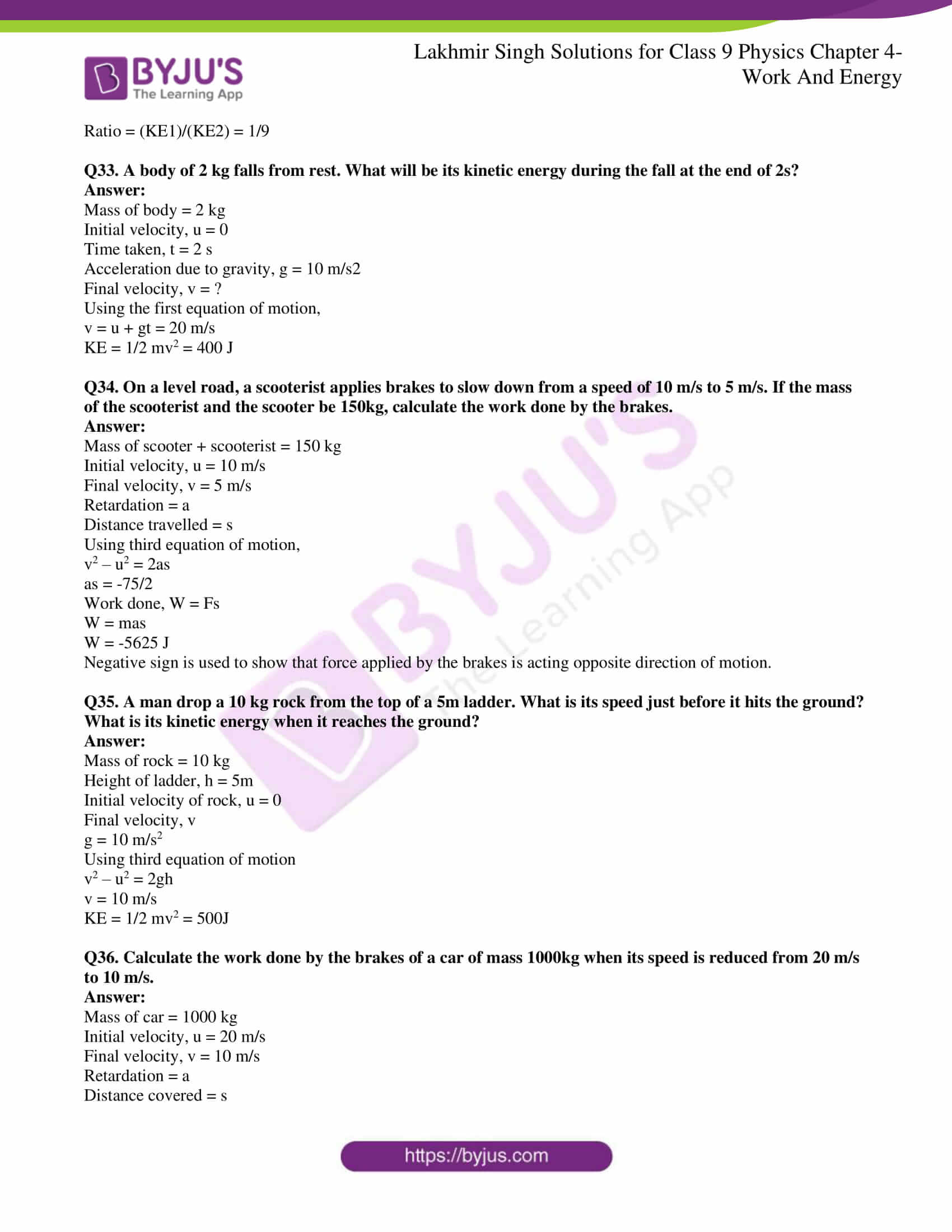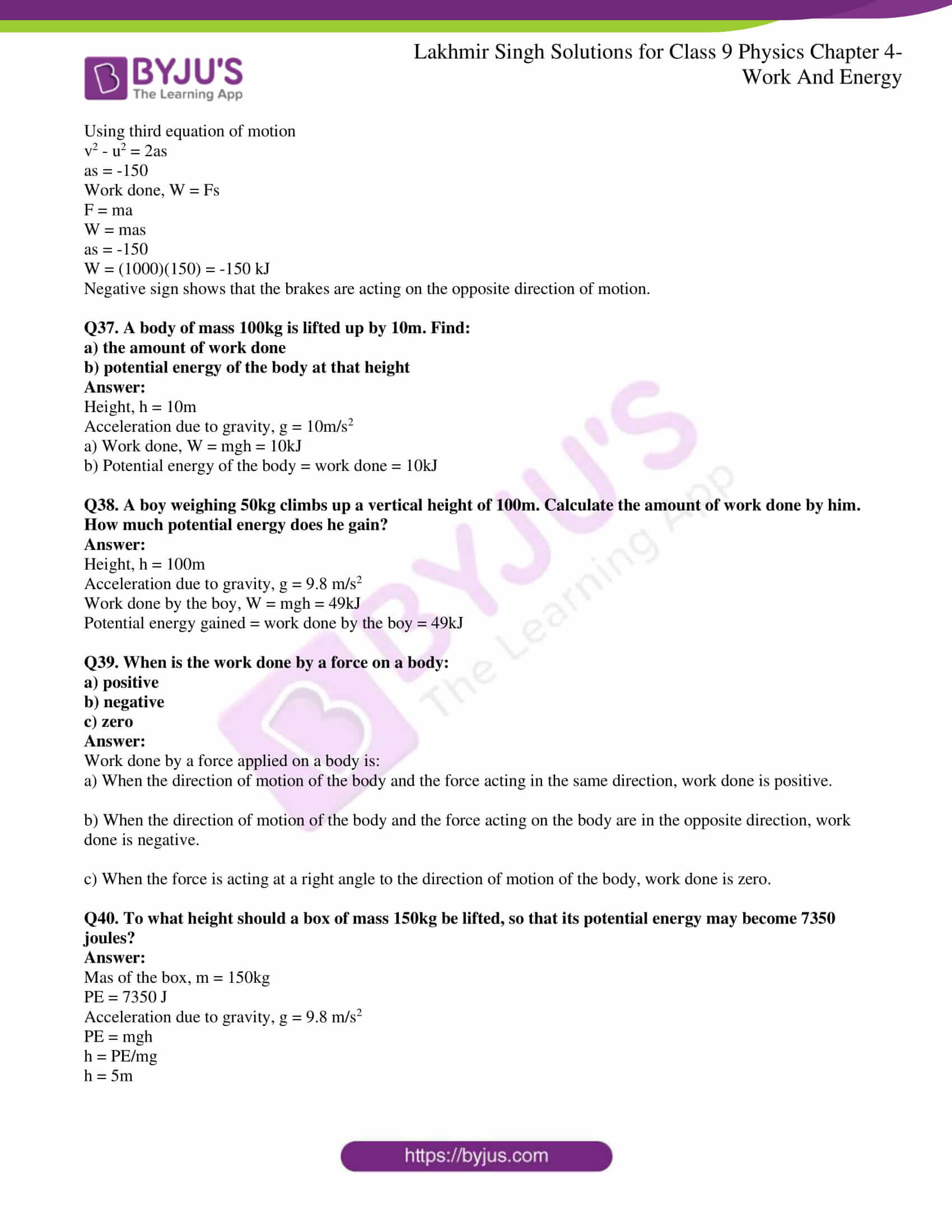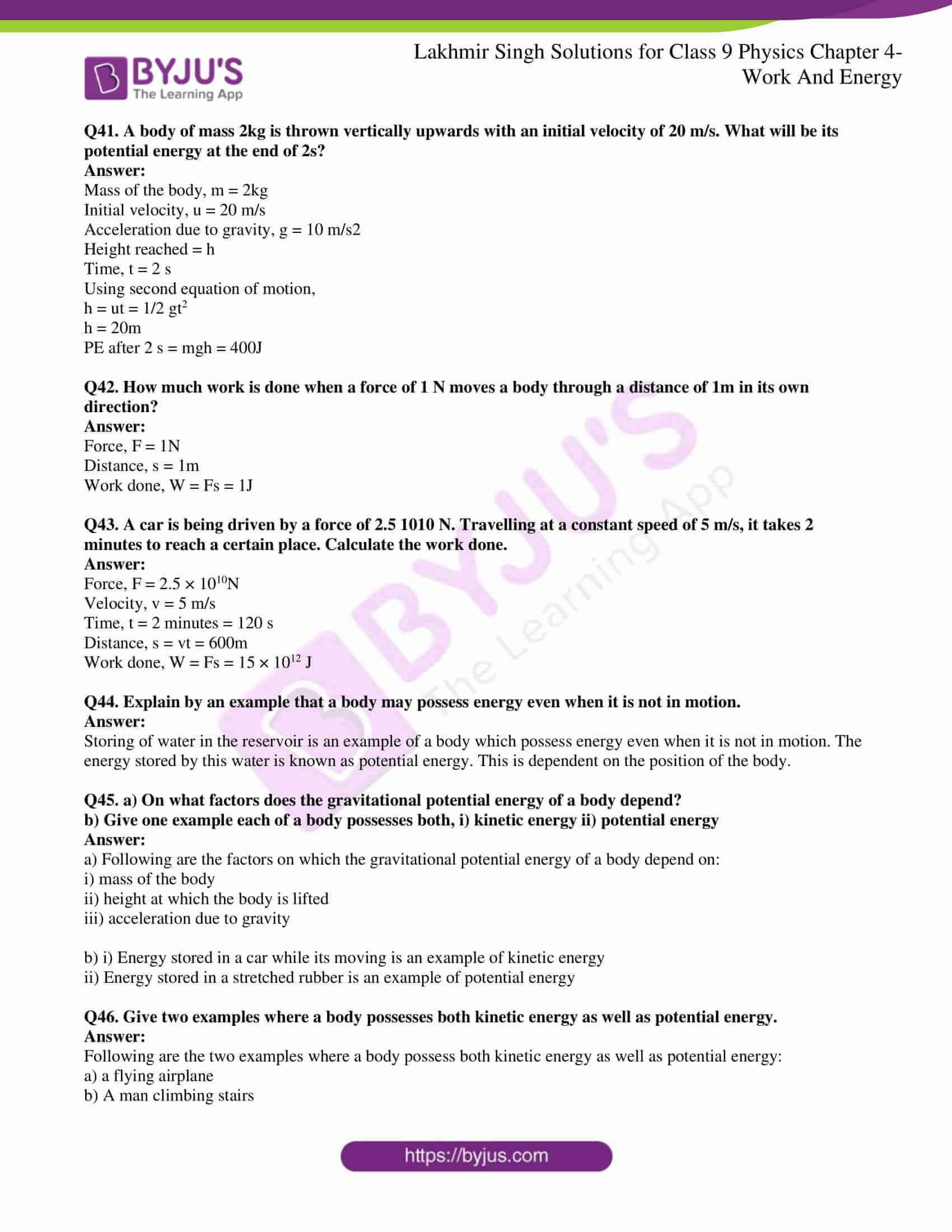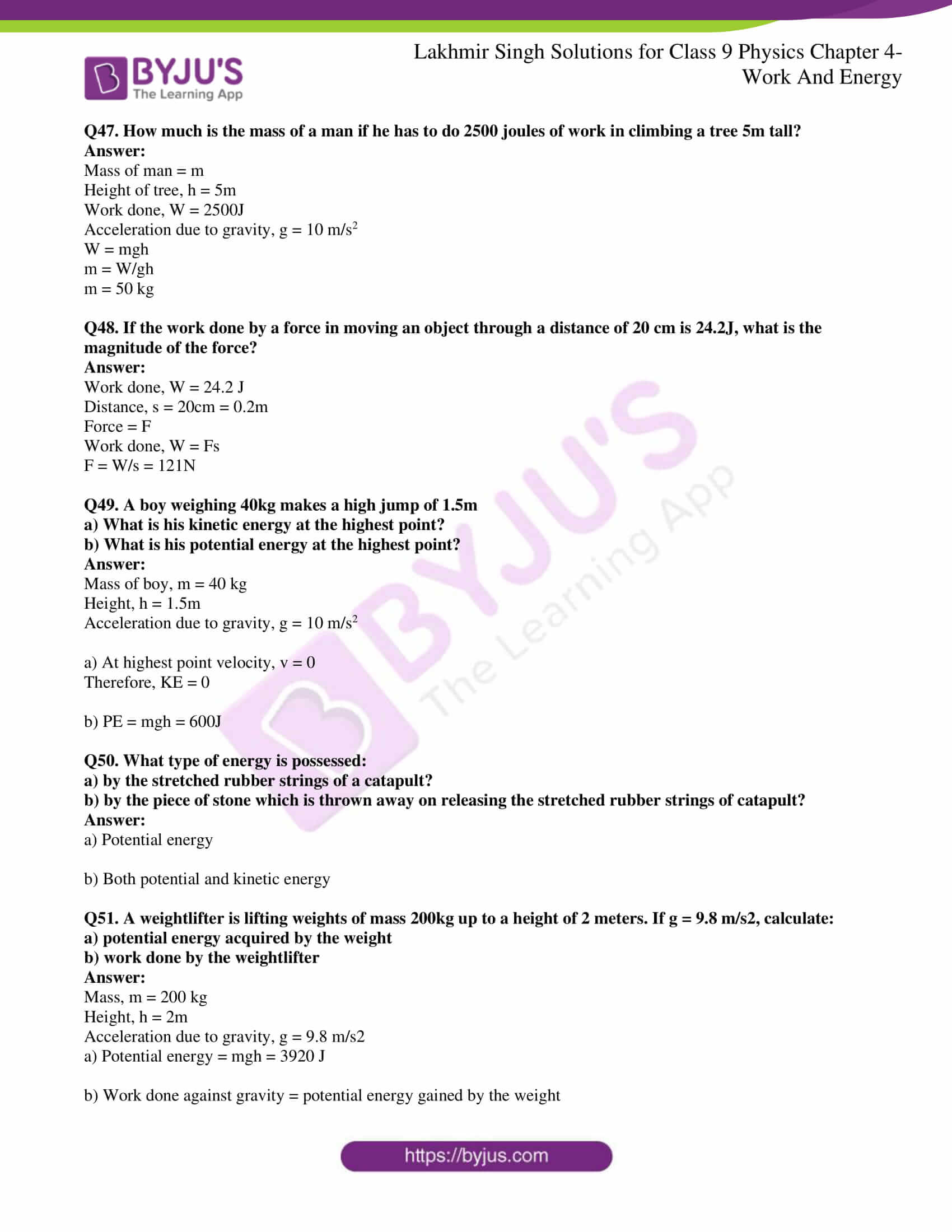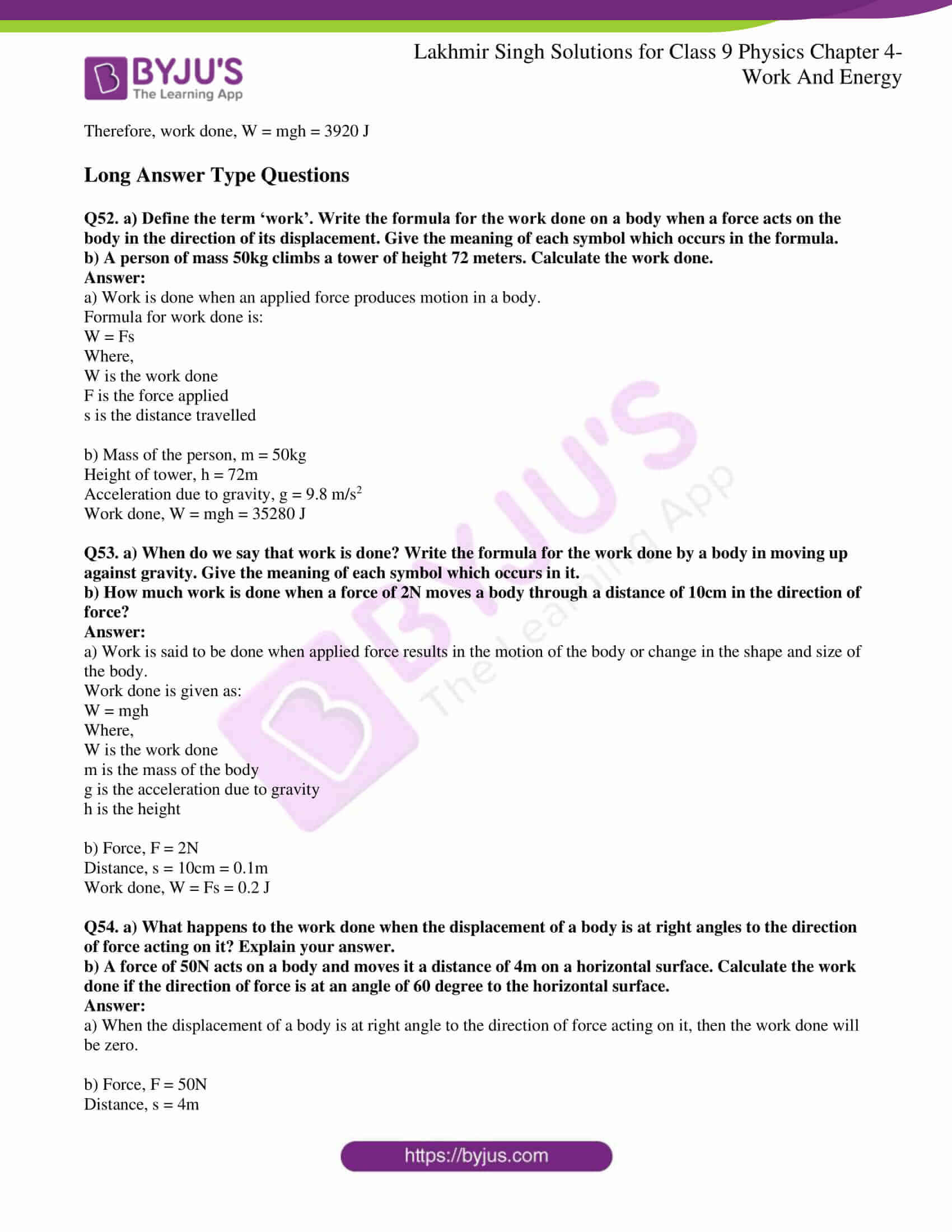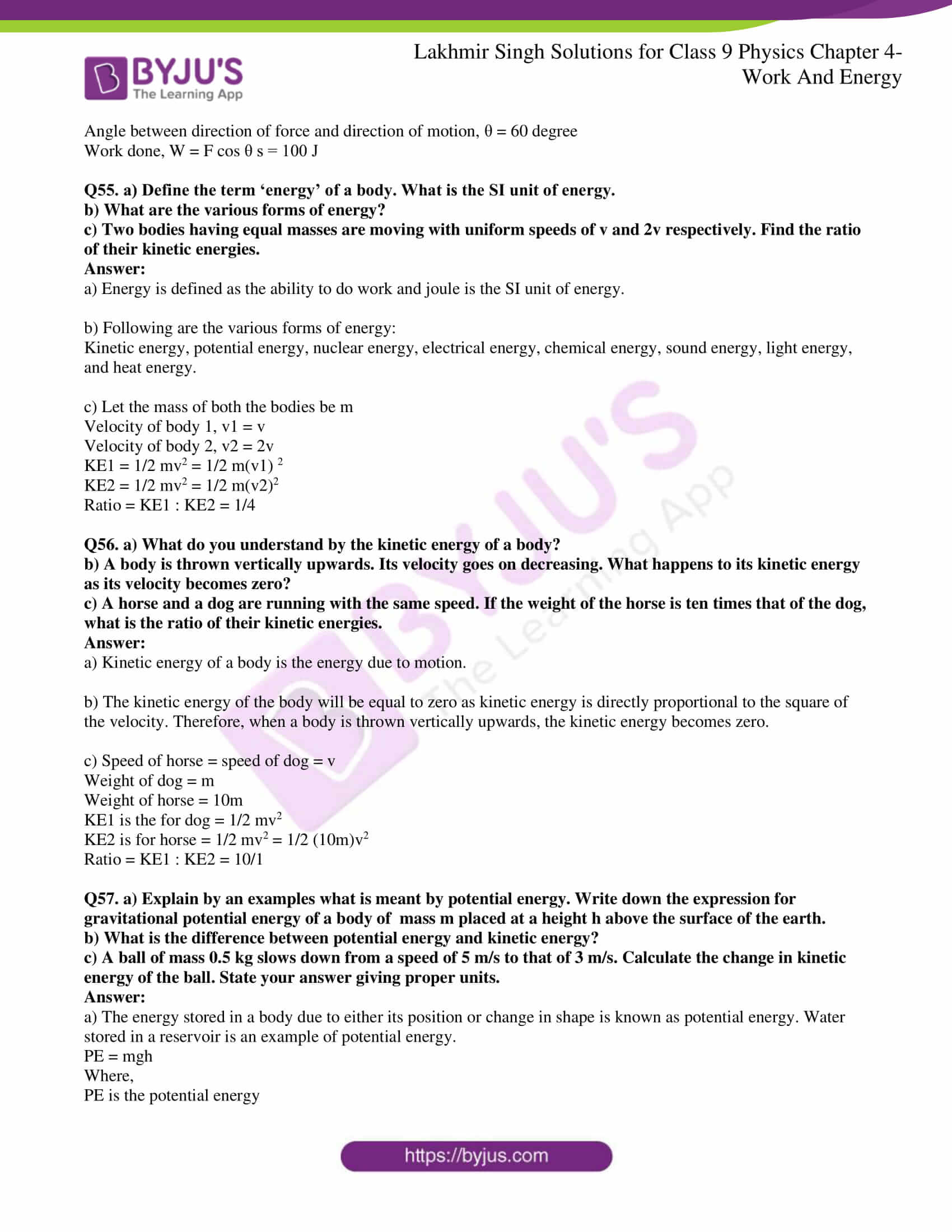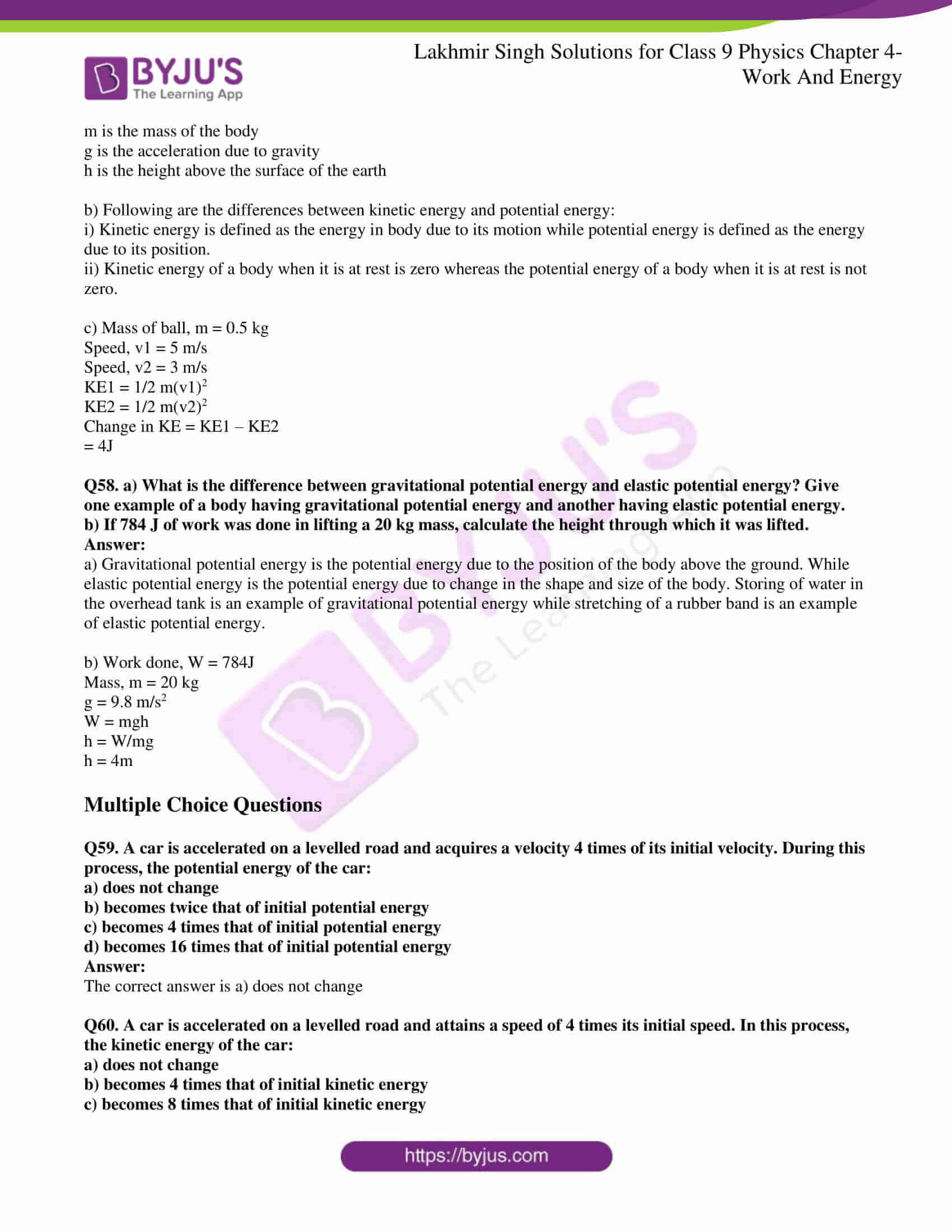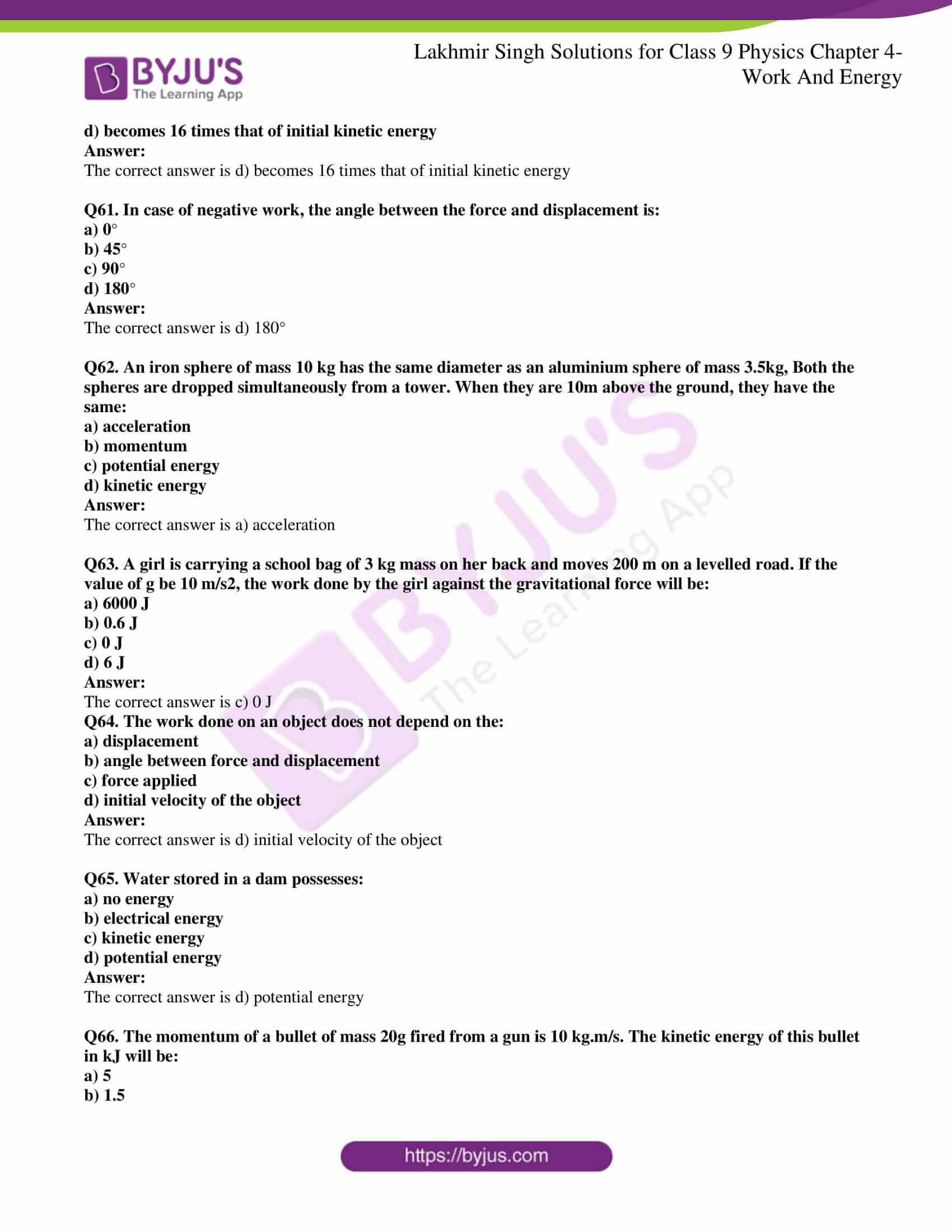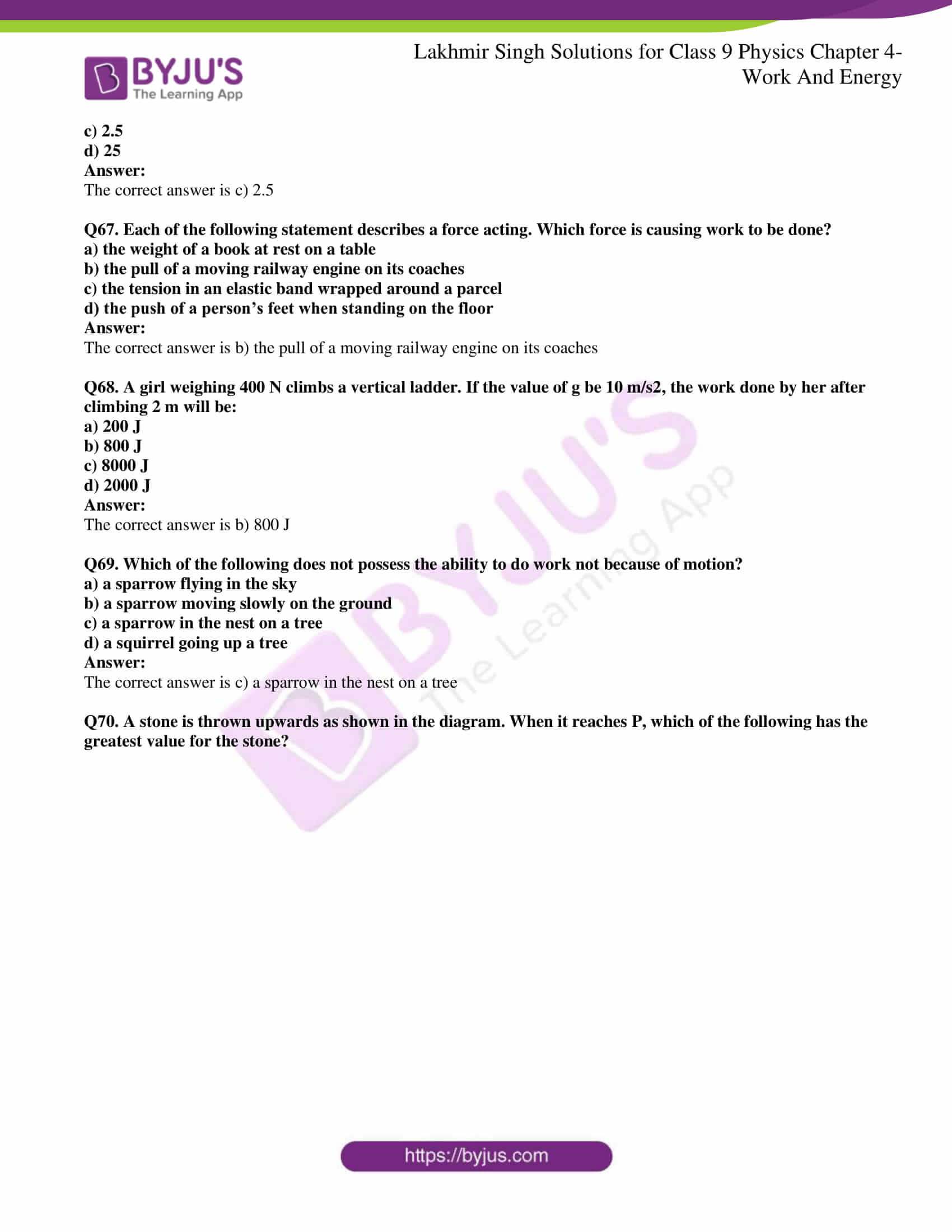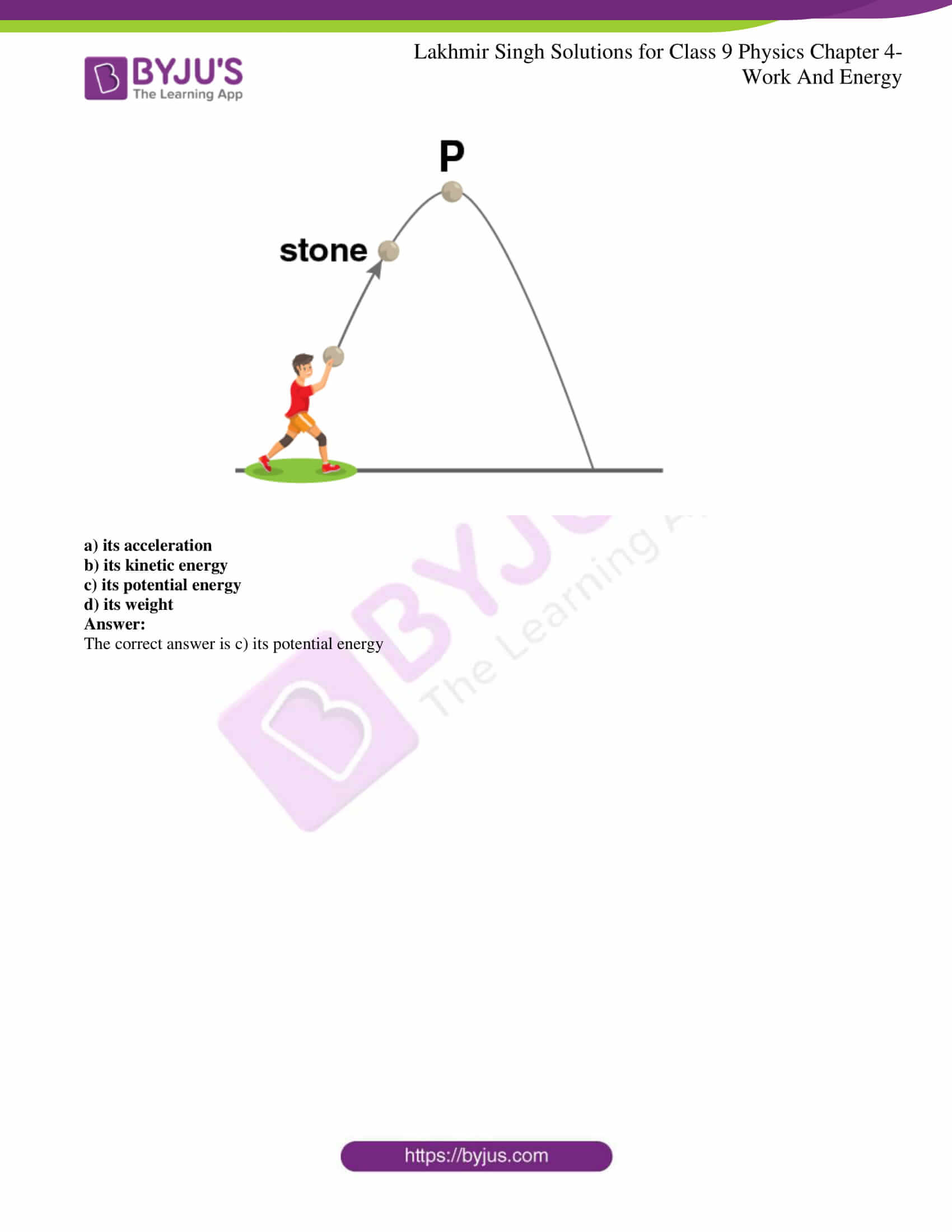Page No: 145

### Very Short Answer Type Questions

Q1. How much work is done when a body of mass m is raised to a height h above the ground?

Mass = m

Height above the ground = h

Work done = potential energy acquired by the body = mgh

Where g is the acceleration due to gravity.

Q2. State the SI unit of work.

SI unit of work is Joule.

Q3. Is work a scalar or a vector quantity?

Work is a scalar quantity as it requires only magnitude.

Q4. Define 1 joule of work.

The work done on a body is said to be 1J when a force of 1N is applied to move a body through a distance of 1m.

Q5. What is the condition for a force to do work on a body?

When a motion is produced in the body, work is said to be done on the body as force is applied on it.

Q6. Is energy a vector quantity?

Energy is a scalar quantity as it has only magnitude.

Q7. What are the units of a) work b) energy

a) Unit of work is Joule

b) Unit of energy is Joule.

Q8. What is the work done against gravity when a body is moved horizontally along a frictionless surface?

The work done against the gravity when a body is move horizontally along a frictionless surface is zero as the force of gravity acts perpendicular to the direction of motion.

Q9. By how much will the kinetic energy of a body increase if its speed is doubled?

The kinetic energy is directly proportional to the square of the speed of the body. Therefore, when the kinetic energy of the body is increased, the kinetic energy will become four times the actual value.

Q10. Write an expression for the kinetic energy of a body of mass m moving with a velocity v.

Mass = m

Velocity = v

KE = ½ mv2

Q11. If the speed of a body is halved, what will be the change in its kinetic energy?

The kinetic energy will become one-fourth the actual value when the speed of the body is halved.

Q12. On what factors does the kinetic energy of a body depend?

Following are the factors on which the kinetic energy of a body depends:

a) mass of the body, m.

b) square of velocity of the body, v2.

Q13. Which would have a greater effect on the kinetic energy of an object: doubling the mass or doubling the velocity?

Doubling the velocity would have a greater effect on the kinetic energy of an object.

Q14. How fast should a man of 50 kg run so that his kinetic energy will be 625J?

Mass = 50kg

Kinetic energy = 625 J

v = ?

KE = ½ mv2

v = 5 m/s

Q15. State whether the following objects possess kinetic energy, potential energy, or both:

a) a man climbing a hill

b) a flying aeroplane

c) a bird running on the ground

d) a ceiling fan in the off position

e) a stretched spring lying on the ground

a) Both kinetic and potential energy

b) Both kinetic and potential energy

c) Only kinetic energy

d) Only potential energy

e) Only potential energy

Q16. Two bodies A and B of equal masses are kept at heights of h and 2h respectively. What will be the ratio of their potential energies?

Let the masses of A and B = m

Height of A = h

Height of B = 2h

Potential energy for body A, PEA = mgh

Potential energy for body B, PEB = mg2h

Ratio = PEA : PEB = (mgh)/(mg2h) = ½

Q17. What is the kinetic energy of a body of mass 1 kg moving with a speed of 2 m/s?

Mass = 1 kg

Velocity = 2 m/s

KE = ½ mv2 = 2J

Q18. Is potential energy a vector or a scalar quantity?

Potential energy is a scalar quantity as it has magnitude only.

Q19. A load of 100 kg is pulled up by 5 m. Calculate the work done.

Mass = 100 kg

Height = 5 m

g = 9.8 m/s2

PE = mgh = 4900J

Work done = potential energy

Q20. State whether the following statement is true or false:

The potential energy of a body of mass 1 kg kept at a height of 1m is 1J.

False

PE = mgh

= (1) (9.8) (1)

= 9.8J

Q21. What happens to the potential energy of a body when its height is doubled?

Potential energy is directly proportional to the height. Therefore, when the height is doubled, potential energy also gets doubled.

Q22. What kind of energy is possessed by the following:

a) a stone kept on roof-top

b) a running car

c) water stored in the reservoir of a dam

d) a compressed spring

e) a stretched rubber band.

a) Potential energy

b) Kinetic energy

c) Potential energy

d) Potential energy

e) Potential energy

Q23. Fill in the blanks with suitable words:

a) Work is measured as a product of ………. and ………..

b) The work done on a body moving in a circular path is ……………

c) 1 joule is the work done when a force of one ………….. moves an object through a distance of one ……….. in the direction of ………..

d) The ability of a body to do work is called ……….. The ability of a body to do work because of its motion is called ………..

e) The sum of the potential and kinetic energies of a body is called ………….. energy.

a) Force, distance

b) Zero

c) Newton, meter, force

d) Energy, kinetic energy

e) Mechanical

Q24. What are the quantities on which the amount of work done depends? How are they related to work?

Following are the quantities on which the amount of work done depends:

a) magnitude of the force

b) distance through which the body moves

Force and distance are related to work done as work done is directly proportional to the force applied and the distance moved by the body.

W = Fs

Where,

W is the work done

F is the force applied

s is the distance travelled

Q25. Is it possible that a force is acting on a body but still the work done is zero? Explain giving one example.

Yes, it is possible that a force is acting on a body and still the work done is zero.

When a man is pushing a wall, work done is zero as there is no displacement.

Q26. A boy throws a rubber ball vertically upwards. What type of work, positive or negative is done:

a) by the force applied by the boy?

b) by the gravitational force of earth?

a) The force applied by the boy is a positive work as it is in the direction of motion of the body.

b) The work done by the gravitational force of the earth is negative as it is against the direction of motion of the body.

Q27. Write the formula for work done on a body when the body moves at an angle to the direction of force. Give the meaning of each symbol used.

W = F cos θ s

Where,

W is the work done

F is the force applied

s is the distance travelled by the body

θ is the angle between the direction of force and the direction of motion of the body

Q28. How does the kinetic energy of a moving body depend on its

a) speed

b) mass

a) Kinetic energy is directly proportional to the speed.

b) Kinetic energy is directly proportional to the mass.

Q29. Give one example each in which a force does

a) positive work

b) negative work

c) zero work

a) Positive work: When a ball is thrown upwards, work done by the force is positive work.

b) Negative work: Work done by the gravitational force of the earth on the ball thrown which is thrown upwards.

c) Zero work: Sliding of the box on the ground is an example of zero work as the gravitational force acting on the box.

Q30. A ball of mass 200g falls from a height of 5 meters. What is its kinetic energy when it just reaches the ground?

Mass = 200 g = 0.2kg

Height = 5m

Initial velocity, u = 0

Acceleration due to gravity, g = 9.8 m/s2

Final velocity, v

Using third equation of motion,

v2 – u2 = 2gs

v2 = 98

Kinetic energy = ½ mv2

Kinetic energy = (½)(0.2)(98) = 9.8J

Q31. Find the momentum of a body of mass 100g having a kinetic energy of 20J.

KE = 20 J

Mass = 100g = 0.1 kg

KE = ½ mv2

v2 = 400

v = 20 m/s

Momentum = mv = 2 kg.m/s

Q32. Two objects having equal masses are moving with uniform velocities of 2 m/s and 6 m/s respectively. Calculate the ratio of their kinetic energies.

Let masses of the two objects be m

v1 = 2 m/s

v2 = 6 m/s

KE1 = 1/2 m.(2)2

KE2 = 1/2 m.(6)2

Ratio = (KE1)/(KE2) = 1/9

Q33. A body of 2 kg falls from rest. What will be its kinetic energy during the fall at the end of 2s?

Mass of body = 2 kg

Initial velocity, u = 0

Time taken, t = 2 s

Acceleration due to gravity, g = 10 m/s2

Final velocity, v = ?

Using the first equation of motion,

v = u + gt = 20 m/s

KE = 1/2 mv2 = 400 J

Q34. On a level road, a scooterist applies brakes to slow down from a speed of 10 m/s to 5 m/s. If the mass of the scooterist and the scooter be 150kg, calculate the work done by the brakes.

Mass of scooter + scooterist = 150 kg

Initial velocity, u = 10 m/s

Final velocity, v = 5 m/s

Retardation = a

Distance travelled = s

Using third equation of motion,

v2 – u2 = 2as

as = -75/2

Work done, W = Fs

W = mas

W = -5625 J

Negative sign is used to show that force applied by the brakes is acting opposite direction of motion.

Q35. A man drop a 10 kg rock from the top of a 5m ladder. What is its speed just before it hits the ground? What is its kinetic energy when it reaches the ground?

Mass of rock = 10 kg

Height of ladder, h = 5m

Initial velocity of rock, u = 0

Final velocity, v

g = 10 m/s2

Using third equation of motion

v2 – u2 = 2gh

v = 10 m/s

KE = 1/2 mv2 = 500J

Q36. Calculate the work done by the brakes of a car of mass 1000kg when its speed is reduced from 20 m/s to 10 m/s.

Mass of car = 1000 kg

Initial velocity, u = 20 m/s

Final velocity, v = 10 m/s

Retardation = a

Distance covered = s

Using third equation of motion

v2 – u2 = 2as

as = -150

Work done, W = Fs

F = ma

W = mas

as = -150

W = (1000)(150) = -150 kJ

Negative sign shows that the brakes are acting on the opposite direction of motion.

Q37. A body of mass 100kg is lifted up by 10m. Find:

a) the amount of work done

b) potential energy of the body at that height

Height, h = 10m

Acceleration due to gravity, g = 10m/s2

a) Work done, W = mgh = 10kJ

b) Potential energy of the body = work done = 10kJ

Q38. A boy weighing 50kg climbs up a vertical height of 100m. Calculate the amount of work done by him. How much potential energy does he gain?

Height, h = 100m

Acceleration due to gravity, g = 9.8 m/s2

Work done by the boy, W = mgh = 49kJ

Potential energy gained = work done by the boy = 49kJ

Q39. When is the work done by a force on a body:

a) positive

b) negative

c) zero

Work done by a force applied on a body is:

a) When the direction of motion of the body and the force acting in the same direction, work done is positive.

b) When the direction of motion of the body and the force acting on the body are in the opposite direction, work done is negative.

c) When the force is acting at a right angle to the direction of motion of the body, work done is zero.

Q40. To what height should a box of mass 150kg be lifted, so that its potential energy may become 7350 joules?

Mas of the box, m = 150kg

PE = 7350 J

Acceleration due to gravity, g = 9.8 m/s2

PE = mgh

h = PE/mg

h = 5m

Q41. A body of mass 2kg is thrown vertically upwards with an initial velocity of 20 m/s. What will be its potential energy at the end of 2s?

Mass of the body, m = 2kg

Initial velocity, u = 20 m/s

Acceleration due to gravity, g = 10 m/s2

Height reached = h

Time, t = 2 s

Using second equation of motion,

h = ut = 1/2 gt2

h = 20m

PE after 2 s = mgh = 400J

Q42. How much work is done when a force of 1 N moves a body through a distance of 1m in its own direction?

Force, F = 1N

Distance, s = 1m

Work done, W = Fs = 1J

Q43. A car is being driven by a force of 2.5 1010 N. Travelling at a constant speed of 5 m/s, it takes 2 minutes to reach a certain place. Calculate the work done.

Force, F = 2.5 × 1010N

Velocity, v = 5 m/s

Time, t = 2 minutes = 120 s

Distance, s = vt = 600m

Work done, W = Fs = 15 × 1012 J

Q44. Explain by an example that a body may possess energy even when it is not in motion.

Storing of water in the reservoir is an example of a body which possess energy even when it is not in motion. The energy stored by this water is known as potential energy. This is dependent on the position of the body.

Q45. a) On what factors does the gravitational potential energy of a body depend?

b) Give one example each of a body possesses both, i) kinetic energy ii) potential energy

a) Following are the factors on which the gravitational potential energy of a body depend on:

i) mass of the body

ii) height at which the body is lifted

iii) acceleration due to gravity

b) i) Energy stored in a car while its moving is an example of kinetic energy

ii) Energy stored in a stretched rubber is an example of potential energy

Q46. Give two examples where a body possesses both kinetic energy as well as potential energy.

Following are the two examples where a body possess both kinetic energy as well as potential energy:

a) a flying airplane

b) A man climbing stairs

Q47. How much is the mass of a man if he has to do 2500 joules of work in climbing a tree 5m tall?

Mass of man = m

Height of tree, h = 5m

Work done, W = 2500J

Acceleration due to gravity, g = 10 m/s2

W = mgh

m = W/gh

m = 50 kg

Q48. If the work done by a force in moving an object through a distance of 20 cm is 24.2J, what is the magnitude of the force?

Work done, W = 24.2 J

Distance, s = 20cm = 0.2m

Force = F

Work done, W = Fs

F = W/s = 121N

Q49. A boy weighing 40kg makes a high jump of 1.5m

a) What is his kinetic energy at the highest point?

b) What is his potential energy at the highest point?

Mass of boy, m = 40 kg

Height, h = 1.5m

Acceleration due to gravity, g = 10 m/s2

a) At highest point velocity, v = 0

Therefore, KE = 0

b) PE = mgh = 600J

Q50. What type of energy is possessed:

a) by the stretched rubber strings of a catapult?

b) by the piece of stone which is thrown away on releasing the stretched rubber strings of catapult?

a) Potential energy

b) Both potential and kinetic energy

Q51. A weightlifter is lifting weights of mass 200kg up to a height of 2 meters. If g = 9.8 m/s2, calculate:

a) potential energy acquired by the weight

b) work done by the weightlifter

Mass, m = 200 kg

Height, h = 2m

Acceleration due to gravity, g = 9.8 m/s2

a) Potential energy = mgh = 3920 J

b) Work done against gravity = potential energy gained by the weight

Therefore, work done, W = mgh = 3920 J

Q52. a) Define the term ‘work’. Write the formula for the work done on a body when a force acts on the body in the direction of its displacement. Give the meaning of each symbol which occurs in the formula.

b) A person of mass 50kg climbs a tower of height 72 meters. Calculate the work done.

a) Work is done when an applied force produces motion in a body.

Formula for work done is:

W = Fs

Where,

W is the work done

F is the force applied

s is the distance travelled

b) Mass of the person, m = 50kg

Height of tower, h = 72m

Acceleration due to gravity, g = 9.8 m/s2

Work done, W = mgh = 35280 J

Q53. a) When do we say that work is done? Write the formula for the work done by a body in moving up against gravity. Give the meaning of each symbol which occurs in it.

b) How much work is done when a force of 2N moves a body through a distance of 10cm in the direction of force?

a) Work is said to be done when applied force results in the motion of the body or change in the shape and size of the body.

Work done is given as:

W = mgh

Where,

W is the work done

m is the mass of the body

g is the acceleration due to gravity

h is the height

b) Force, F = 2N

Distance, s = 10cm = 0.1m

Work done, W = Fs = 0.2 J

Q54. a) What happens to the work done when the displacement of a body is at right angles to the direction of force acting on it? Explain your answer.

b) A force of 50N acts on a body and moves it a distance of 4m on a horizontal surface. Calculate the work done if the direction of force is at an angle of 60 degree to the horizontal surface.

a) When the displacement of a body is at right angle to the direction of force acting on it, then the work done will be zero.

b) Force, F = 50N

Distance, s = 4m

Angle between direction of force and direction of motion, θ = 60 degree

Work done, W = F cos θ s = 100 J

Q55. a) Define the term ‘energy’ of a body. What is the SI unit of energy.

b) What are the various forms of energy?

c) Two bodies having equal masses are moving with uniform speeds of v and 2v respectively. Find the ratio of their kinetic energies.

a) Energy is defined as the ability to do work and joule is the SI unit of energy.

b) Following are the various forms of energy:

Kinetic energy, potential energy, nuclear energy, electrical energy, chemical energy, sound energy, light energy, and heat energy.

c) Let the mass of both the bodies be m

Velocity of body 1, v1 = v

Velocity of body 2, v2 = 2v

KE1 = 1/2 mv2 = 1/2 m(v1) 2

KE2 = 1/2 mv2 = 1/2 m(v2)2

Ratio = KE1 : KE2 = 1/4

Q56. a) What do you understand by the kinetic energy of a body?

b) A body is thrown vertically upwards. Its velocity goes on decreasing. What happens to its kinetic energy as its velocity becomes zero?

c) A horse and a dog are running with the same speed. If the weight of the horse is ten times that of the dog, what is the ratio of their kinetic energies.

a) Kinetic energy of a body is the energy due to motion.

b) The kinetic energy of the body will be equal to zero as kinetic energy is directly proportional to the square of the velocity. Therefore, when a body is thrown vertically upwards, the kinetic energy becomes zero.

c) Speed of horse = speed of dog = v

Weight of dog = m

Weight of horse = 10m

KE1 is the for dog = 1/2 mv2

KE2 is for horse = 1/2 mv2 = 1/2 (10m)v2

Ratio = KE1 : KE2 = 10/1

Q57. a) Explain by an examples what is meant by potential energy. Write down the expression for gravitational potential energy of a body of mass m placed at a height h above the surface of the earth.

b) What is the difference between potential energy and kinetic energy?

c) A ball of mass 0.5 kg slows down from a speed of 5 m/s to that of 3 m/s. Calculate the change in kinetic energy of the ball. State your answer giving proper units.

a) The energy stored in a body due to either its position or change in shape is known as potential energy. Water stored in a reservoir is an example of potential energy.

PE = mgh

Where,

PE is the potential energy

m is the mass of the body

g is the acceleration due to gravity

h is the height above the surface of the earth

b) Following are the differences between kinetic energy and potential energy:

i) Kinetic energy is defined as the energy in body due to its motion while potential energy is defined as the energy due to its position.

ii) Kinetic energy of a body when it is at rest is zero whereas the potential energy of a body when it is at rest is not zero.

c) Mass of ball, m = 0.5 kg

Speed, v1 = 5 m/s

Speed, v2 = 3 m/s

KE1 = 1/2 m(v1)2

KE2 = 1/2 m(v2)2

Change in KE = KE1 – KE2

= 4J

Q58. a) What is the difference between gravitational potential energy and elastic potential energy? Give one example of a body having gravitational potential energy and another having elastic potential energy.

b) If 784 J of work was done in lifting a 20 kg mass, calculate the height through which it was lifted.

a) Gravitational potential energy is the potential energy due to the position of the body above the ground. While elastic potential energy is the potential energy due to change in the shape and size of the body. Storing of water in the overhead tank is an example of gravitational potential energy while stretching of a rubber band is an example of elastic potential energy.

b) Work done, W = 784J

Mass, m = 20 kg

g = 9.8 m/s2

W = mgh

h = W/mg

h = 4m

### Multiple Choice Questions

Q59. A car is accelerated on a levelled road and acquires a velocity 4 times of its initial velocity. During this process, the potential energy of the car:

a) does not change

b) becomes twice that of initial potential energy

c) becomes 4 times that of initial potential energy

d) becomes 16 times that of initial potential energy

The correct answer is a) does not change

Q60. A car is accelerated on a levelled road and attains a speed of 4 times its initial speed. In this process, the kinetic energy of the car:

a) does not change

b) becomes 4 times that of initial kinetic energy

c) becomes 8 times that of initial kinetic energy

d) becomes 16 times that of initial kinetic energy

The correct answer is d) becomes 16 times that of initial kinetic energy

Q61. In case of negative work, the angle between the force and displacement is:

a) 0°

b) 45°

c) 90°

d) 180°

The correct answer is d) 180°

Q62. An iron sphere of mass 10 kg has the same diameter as an aluminium sphere of mass 3.5kg, Both the spheres are dropped simultaneously from a tower. When they are 10m above the ground, they have the same:

a) acceleration

b) momentum

c) potential energy

d) kinetic energy

The correct answer is a) acceleration

Q63. A girl is carrying a school bag of 3 kg mass on her back and moves 200 m on a levelled road. If the value of g be 10 m/s2, the work done by the girl against the gravitational force will be:

a) 6000 J

b) 0.6 J

c) 0 J

d) 6 J

The correct answer is c) 0 J

Q64. The work done on an object does not depend on the:

a) displacement

b) angle between force and displacement

c) force applied

d) initial velocity of the object

The correct answer is d) initial velocity of the object

Q65. Water stored in a dam possesses:

a) no energy

b) electrical energy

c) kinetic energy

d) potential energy

The correct answer is d) potential energy

Q66. The momentum of a bullet of mass 20g fired from a gun is 10 kg.m/s. The kinetic energy of this bullet in kJ will be:

a) 5

b) 1.5

c) 2.5

d) 25

The correct answer is c) 2.5

Q67. Each of the following statement describes a force acting. Which force is causing work to be done?

a) the weight of a book at rest on a table

b) the pull of a moving railway engine on its coaches

c) the tension in an elastic band wrapped around a parcel

d) the push of a person’s feet when standing on the floor

The correct answer is b) the pull of a moving railway engine on its coaches

Q68. A girl weighing 400 N climbs a vertical ladder. If the value of g be 10 m/s2, the work done by her after climbing 2 m will be:

a) 200 J

b) 800 J

c) 8000 J

d) 2000 J

The correct answer is b) 800 J

Q69. Which of the following does not possess the ability to do work not because of motion?

a) a sparrow flying in the sky

b) a sparrow moving slowly on the ground

c) a sparrow in the nest on a tree

d) a squirrel going up a tree

The correct answer is c) a sparrow in the nest on a tree

Q70. A stone is thrown upwards as shown in the diagram. When it reaches P, which of the following has the greatest value for the stone?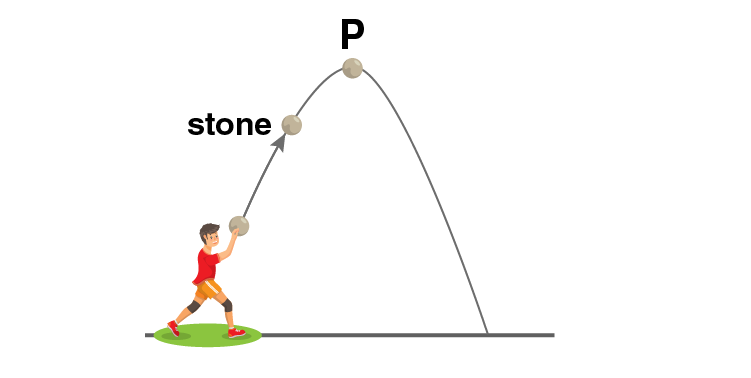a) its acceleration

b) its kinetic energy

c) its potential energy

d) its weight